Date: Mon, 3 Feb 1997 00:36:31 -0600 (CST)
Subject: Deriving the Quadratic Formula

Name: James

Who is asking: Student
Level: Secondary

Question:

How do you derive the quadratic formula? I know what it is, but the textbook doesn't say how to derive it.

Hi James

The quadratic formula is derived by "completing the square". Suppose you have the general quadratic equation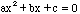Divide both sides by a to get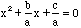or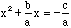By adding the square of one half the coefficient of x to both sides, the left side becomes a square, that is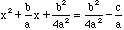or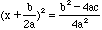.

Thus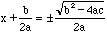,

and finally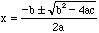Cheers,
Penny

Go to Math Central

To return to the previous page use your browser's back button.